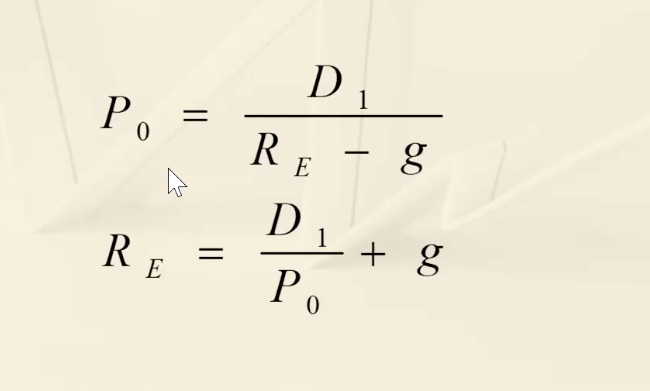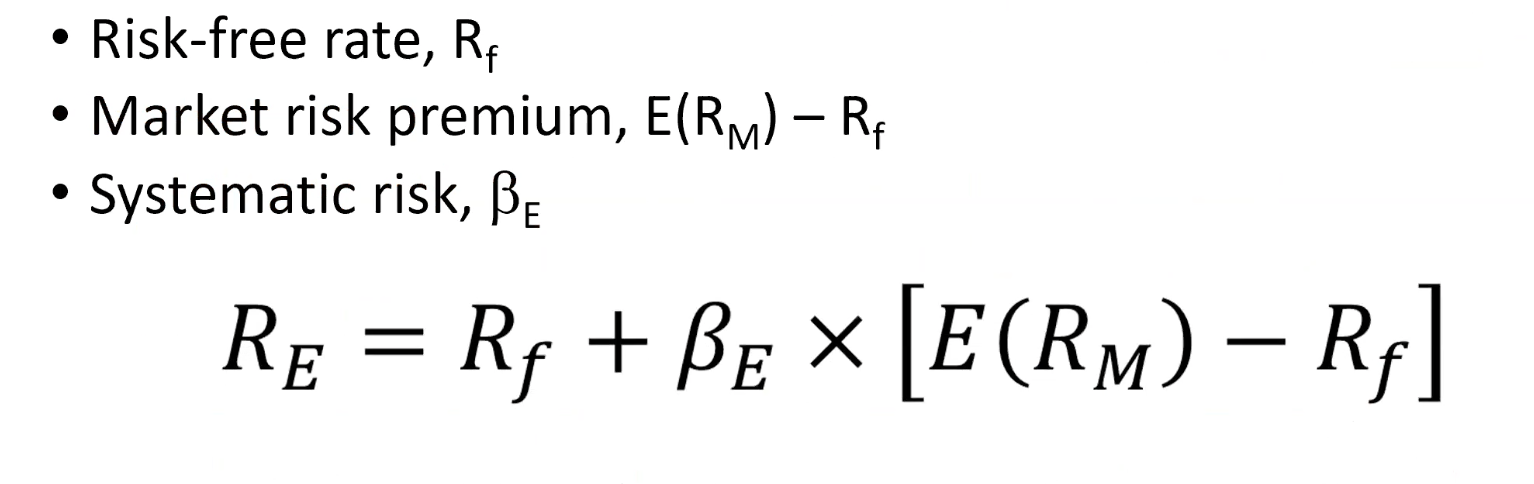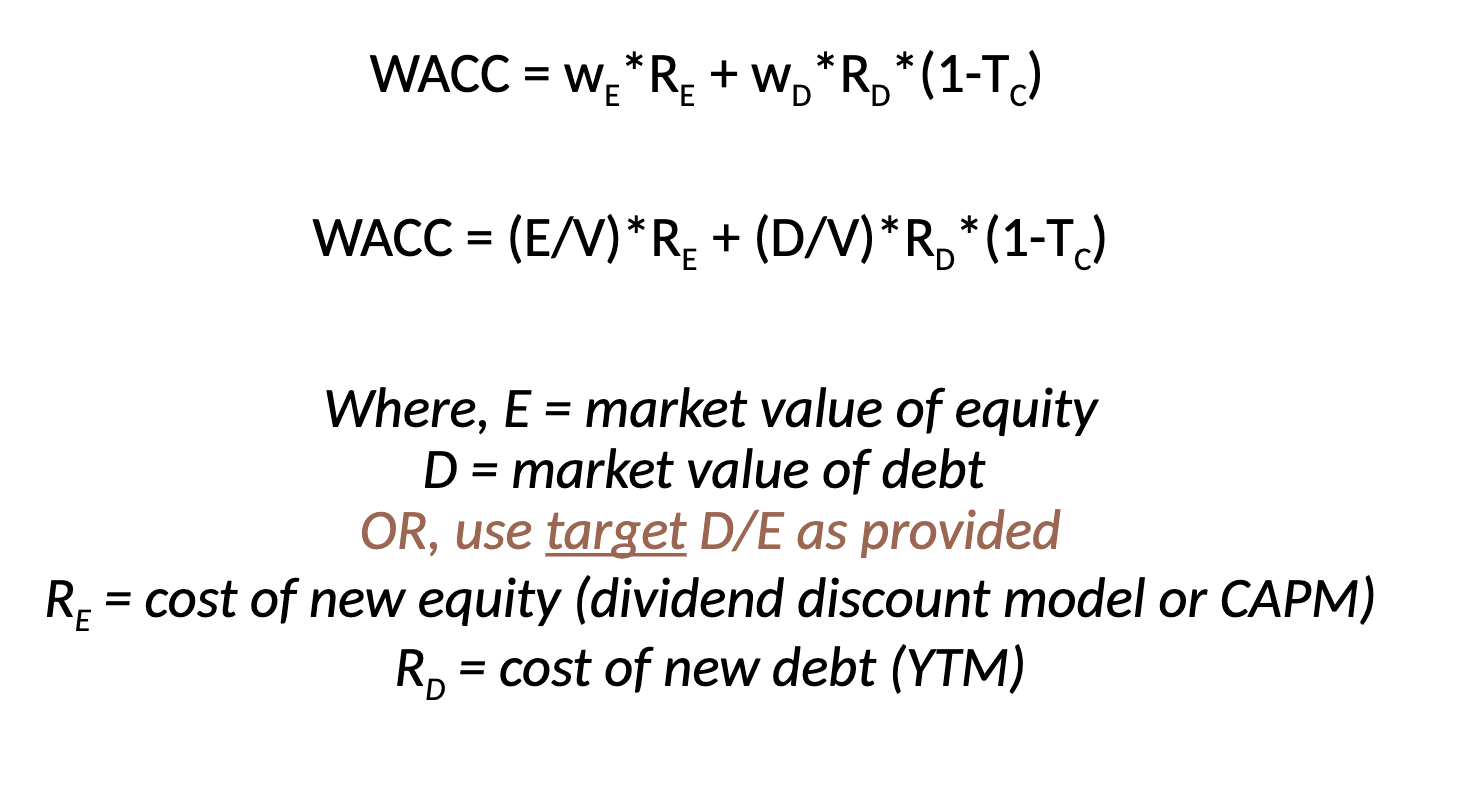# Managerial Finance

Chapter 14

• Importance of cost of capital
• The return earned on assets depends on the risk of those assets
• The return to an investor is the same as the cost to the company
• However, uses of funds (capital budgeting) and sourcing of funds (capital structure) are very separate decisions
• Provides us with an indication of how the market views the risk of the assets
• Knowing the cost of capital can also help determine the required return for capital budgeting projects
• Calculated when company is evaluating a project (not day-to-day)
• Equivalent terms (all mean the same thing)
• Required rate of return
• Cost of capital
• Hurdle rate
• Discount rate
• Cost of equity
• Cost of equity
• Return required by equity investors given the risk of the cash flows from the firm
• Equity investors receive cashflow from the firm in two forms
• Dividends (periodic)
• Selling shares (terminal)
• 2 major methods for determining the cost of equity
• Dividend growth model (DGM)
• SML or CAPM

Dividend Growth Model

• Requirements
• Dividend is expected to grow at a stable rate for the foreseeable future
• Cost of capital > growth of dividend

D1 = next periods dividends, RE = cost of equity, g = growth, P0 = current priceIf D1 is not given: D1 = D0 x (1+g)

*calculating growth:

• Using historical average (new – old)/old = percent change …. average these changes out
• Accounting method:
• 3 assumptions:
• Stable ROE
• Stable dividend policy
• Not planning on raising new external capital
• Then: g = retention ratio x ROE
• Retention ratio = (1-payout ratio)
• Easy to use and understand
• Only applicable to companies currently paying dividends
• Not applicable if dividends aren’t growing at a reasonably constant rate
• Extremely sensitive to the estimated growth rate – increasing g 1% increases cost of equity by 1%
• Does not explicitly consider risk

SML Approach• Market risk premium = market rate – risk free rate
• All equity company**
• Explicitly adjusts for systematic risk
• Applicable to all companies as long as we can compute beta
• Must estimate the expected market risk premium, which varies over time
• Must estimate beta, which also varies over time
• We are relying on the past to predict the future, not always reliable

Note: If 2 models diverge a lot: either average both rates or use SML

• Cost of debt
• Cost of debt is the required return on our company’s debt (Rd)
• Usually focus on the cost of long-term debt or bonds
• Debt investors receive cashflow from the firm in two forms
• Coupon (periodic)
• Selling bond or face/par value (terminal)
• Required return is best estimated by computing the yield-to maturity on the existing bond
• We may also use estimates of current rates based on the bond rating we expect when we issue new debt
• Cost of debt is not the coupon rate (irrelevant)
• ** excel
• Cost of Preferred Stock
• Preferred stocks generally pay a constant dividend every period
• Dividends are expected to be paid every period forever
• Preferred stock is a perpetuity and can be values by: Po = D/Rp
• Rp = D / P0
• D = dividend
• P0 = current price
• Rp = required return – growth

______________________________________________________________________________Weighted Average Cost of Capital

• average” is the required return on assets, based on the market’s perception of the risk of those assets
• The weights are determined by how much of each type of financing is being used – 2 methods:
• Target D/E ratio
• wE = 1 / 1+target D/E
• WD = 1 – wE
• 0r: WD= (target D/E) / (1 + target D/E)
• Market value of debt and equity (weights)
• wD + wE = 1
• Capital structure weights
• Notation
• E = market value of equity = # outstanding shares * price per share
• D = market value of debt = # outstanding bonds * bond price
• V = market value of the firm = D + E
• Target capital structure = D/E
• Weights
• wE = E/V (% financed with equity)
• wD = D/V (%financed with debt)
• Impact of taxes
• We are concerned with after-tax cash flows, so we need to consider the effect of taxes on the various costs of capital
• Interest expense reduced tax liability
• This reduction in taxes reduces cost of debt
• After-tax cost of debt = Rd (1-Tc)
• = Pretax rate*(1-tax rate)
• Tax shield amount = (debt)*(coupon/Rd)*(tax%)
• Dividends are not tax deductible, so there is no tax impact on the cost of equity_____________________________________________________________________________

Divisional and Project Costs of Capital

• Using WACC to evaluate projects only appropriate for projects that have the same risk as the firm’s current operations
• If a project under evaluation does NOT have the same risk as the firm, a different discount rate (not WACC) to be used
• Divisions where the company is involved in different lines of business also require separate discount rates because they have different levels of risk
• Using one WACC to evaluate all projects irrespective of their riskiness will lead the firm to accept more risky projects and reject less risky ones

Solution 1 – Pure Play Approach

• Use of a WACC that is unique to a particular project
• Use WACC of a “peer” group of companies
• Companies that specialize in the project that is being considered
• Compute the beta for each company in peer group, take an average, find the appropriate return for a project of that risk using CAPM
• Often difficult to find pure play companies

Solution 2 – Subjective Approach

• Consider the project’s risk relative to the firm overall
• If the project is riskier than the firm, use a discount rate greater than the firm-wide WACC
• If the project is less risky than the firm, use a discount rate less than the firm-wide WACC
• May result in incorrect acceptance and rejection, but the error rate should be lower than not considering differential risk at all

_____________________________________________________________________________

Company Valuation Using WACC

• Companies are valued using the capital budgeting approach – discounting all cash flows of the firms to find the NPV
• Process: future adjusted cash flows from assets (CFA) are discounting at WACC